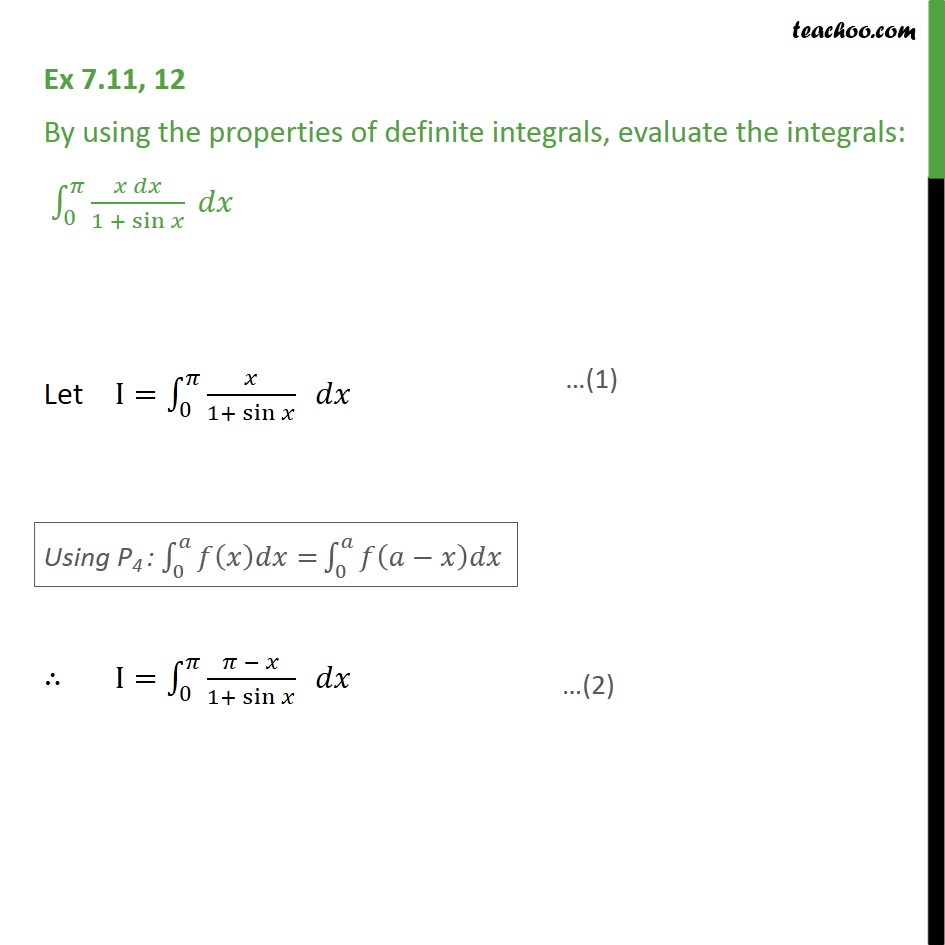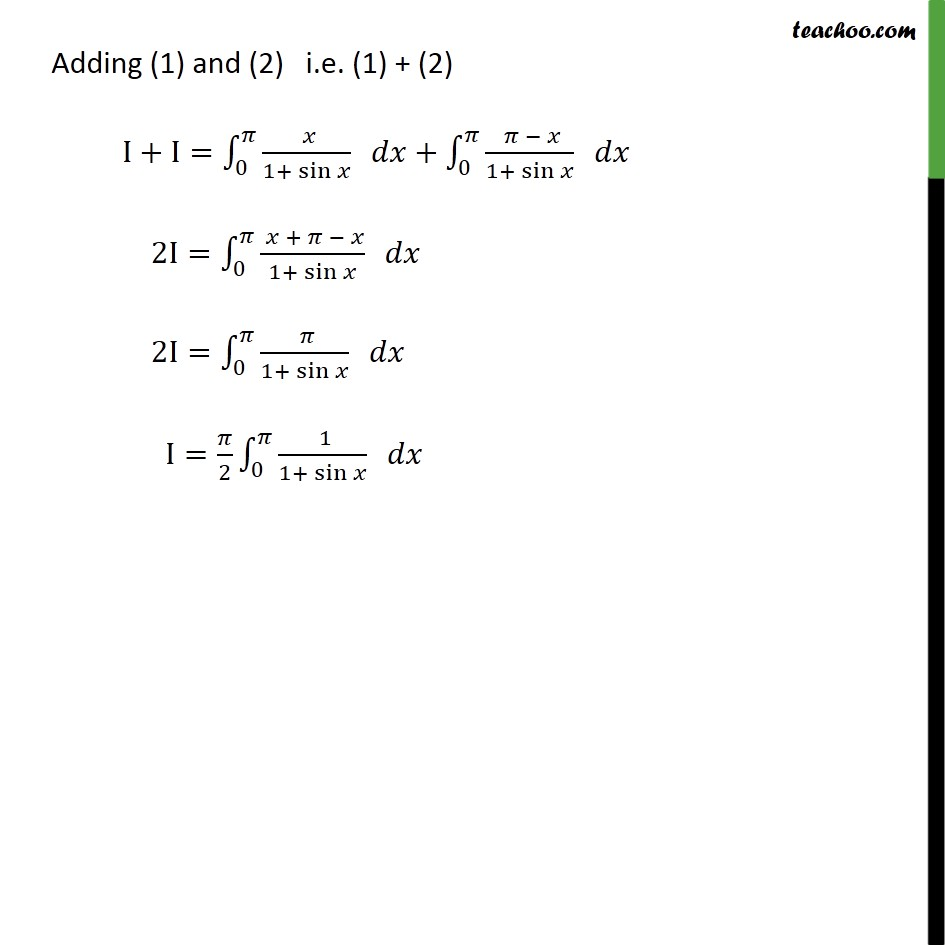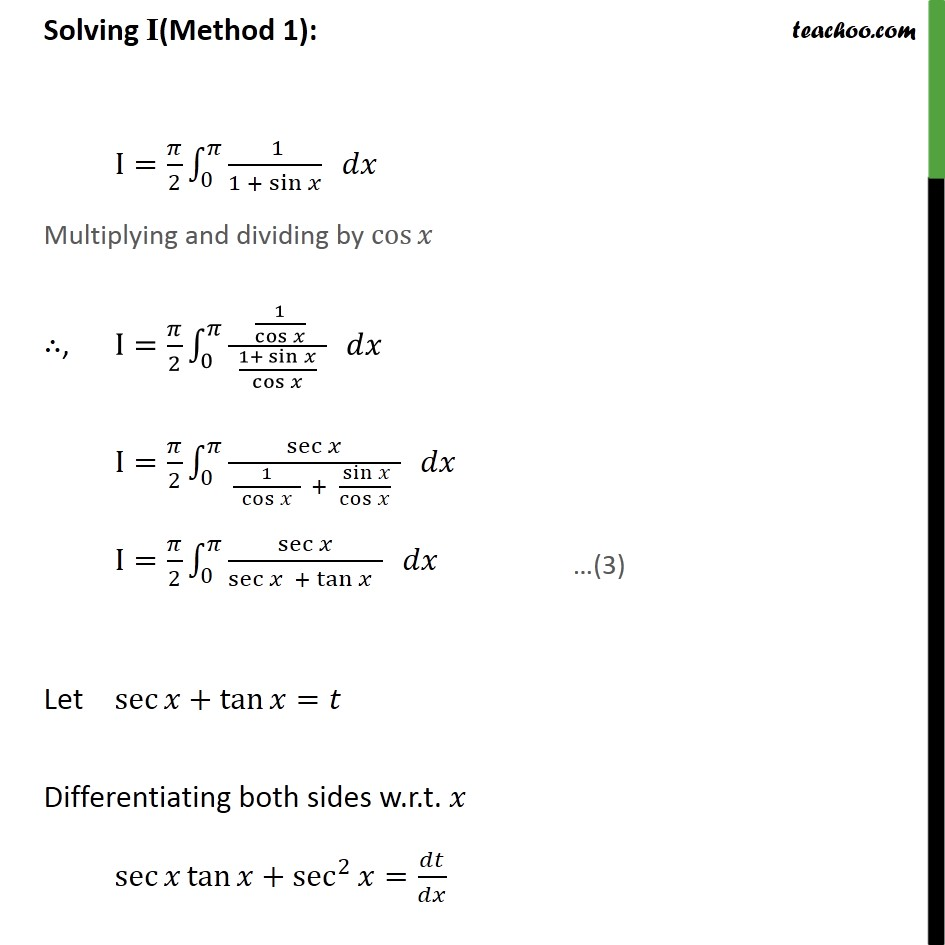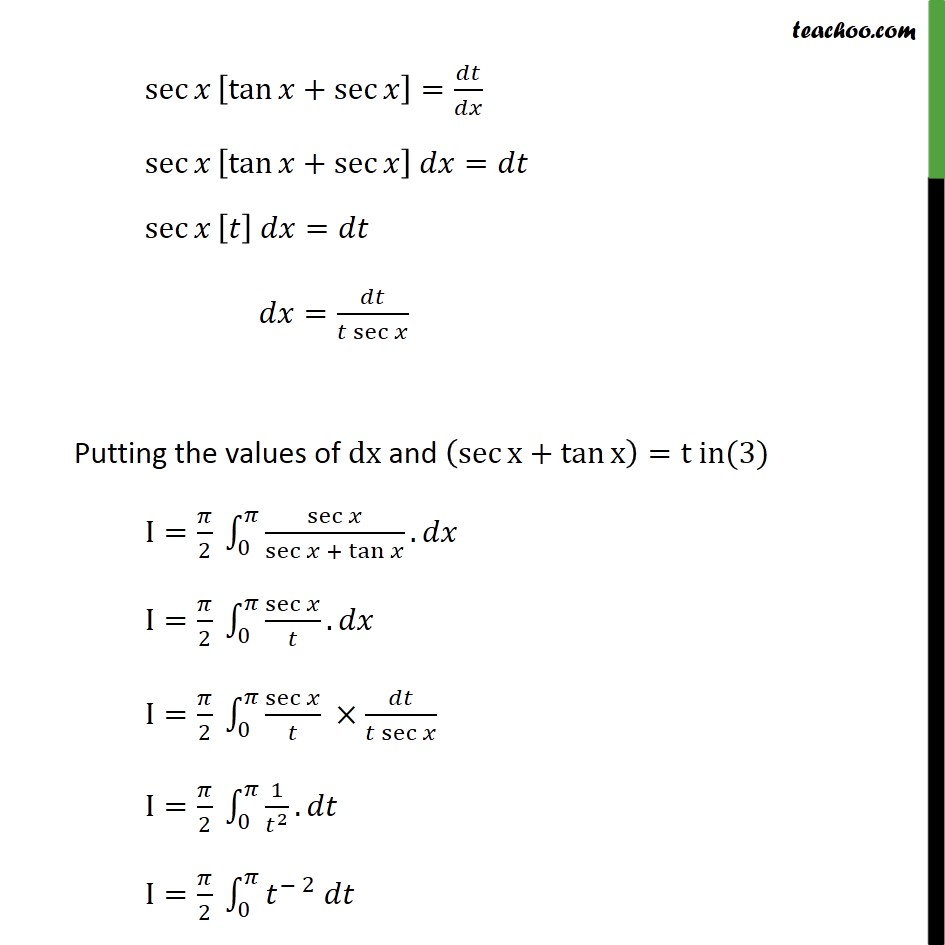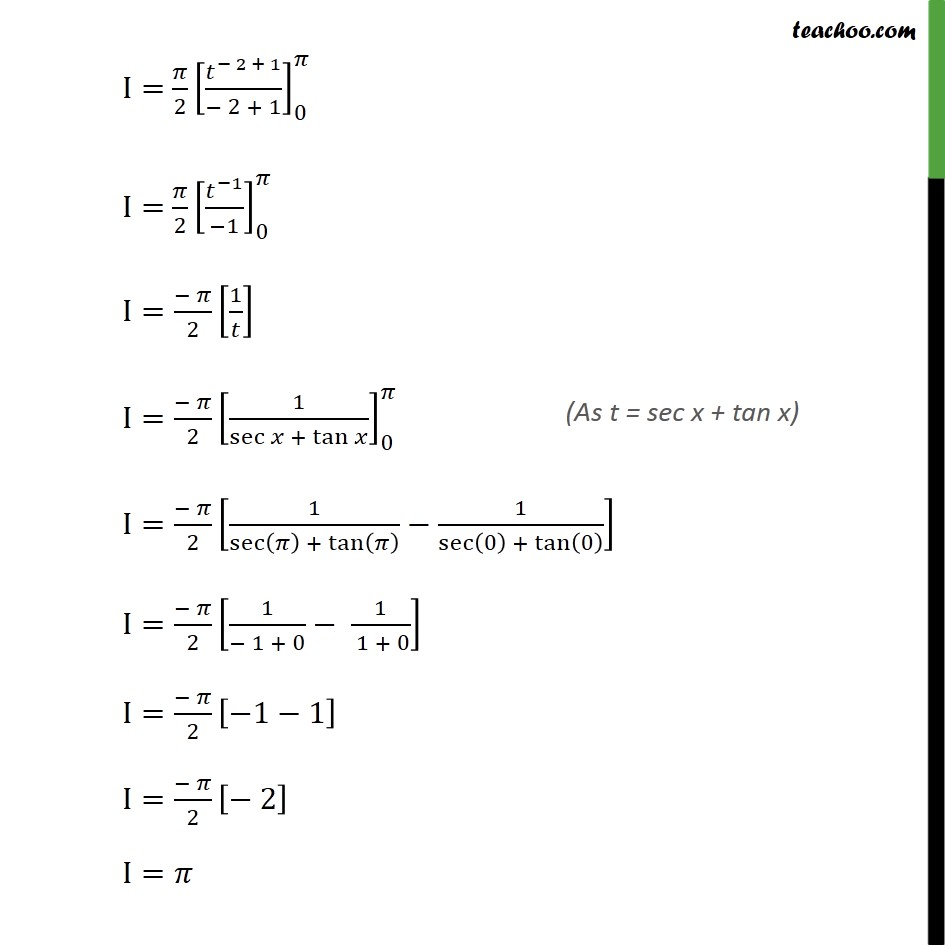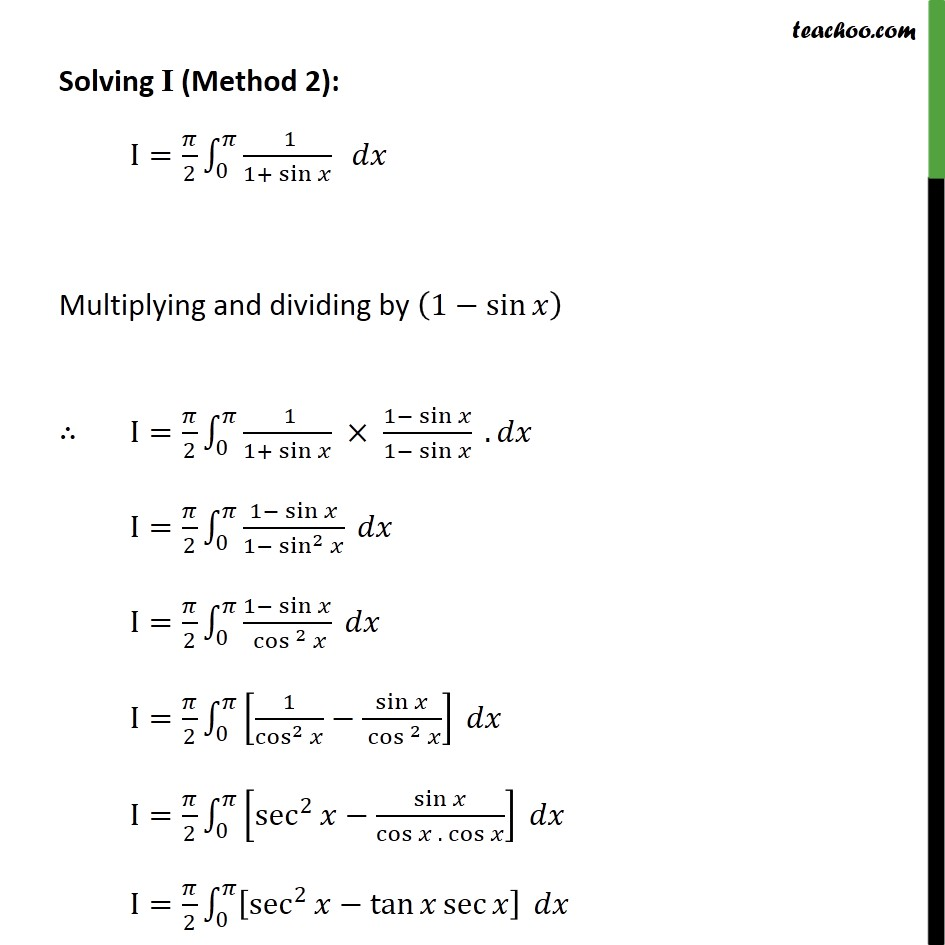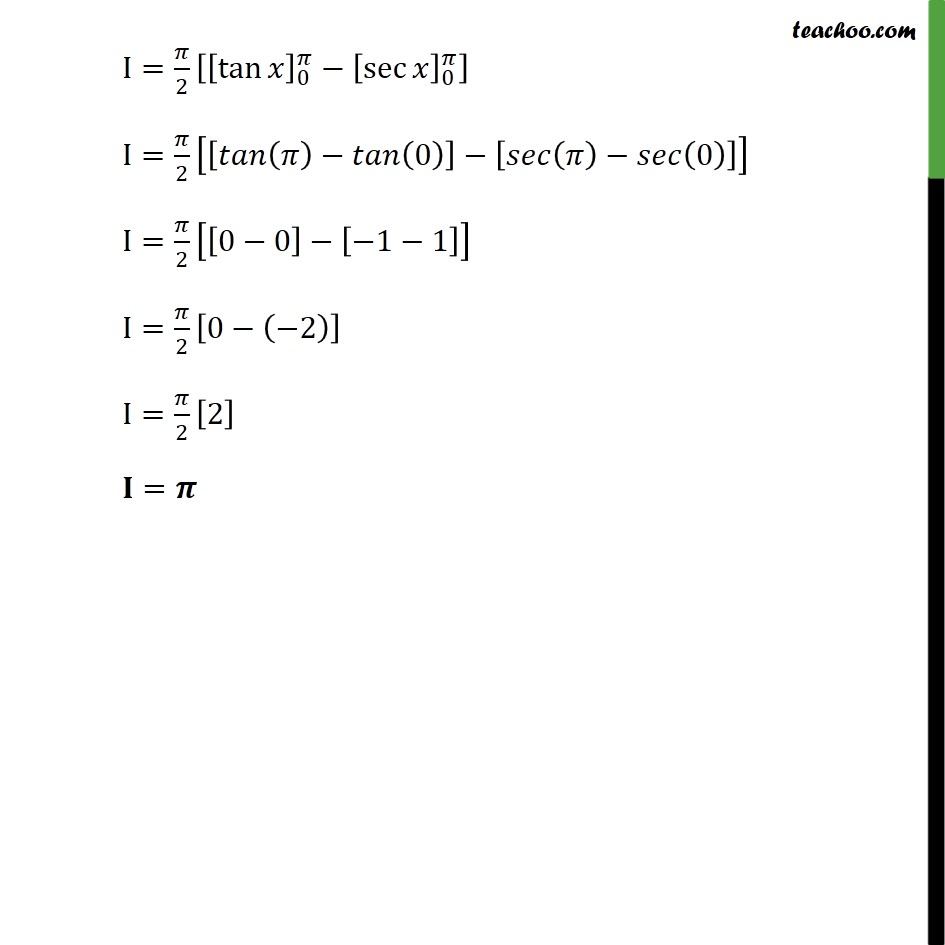1. Chapter 7 Class 12 Integrals
2. Serial order wise
3. Ex 7.11

Transcript

Ex 7.11, 12 By using the properties of definite integrals, evaluate the integrals: _0^ ( )/(1 + sin ) Let I= _0^ /(1+ sin ) I= _0^ ( )/(1+ sin ) Adding (1) and (2) i.e. (1) + (2) I+I= _0^ ( )/(1+ sin ) + _0^ ( )/(1+ sin ) 2I= _0^ ( + )/(1+ sin ) 2I= _0^ ( )/(1+ sin ) I= /2 _0^ ( 1)/(1+ sin ) Solving I(Method 1): I= /2 _0^ ( 1)/(1 + sin ) Multiplying and dividing by cos , I= /2 _0^ ( 1/cos )/( (1+ sin )/cos ) I= /2 _0^ sec /( 1/ cos + ( sin )/cos ) I= /2 _0^ sec /(sec + tan ) Let sec +tan = Differentiating both sides w.r.t. sec tan +sec^2 = / sec [tan +sec ]= / sec [tan +sec ] = sec [ ] = = /( sec ) Putting the values of dx and (sec x+tan x )=t in(3) I= /2 _0^ sec /sec + tan . I= /2 _0^ sec / . I= /2 _0^ sec / /( sec ) I= /2 _0^ 1/ ^2 . I= /2 _0^ ^( 2) I= /2 [ ^( 2 + 1)/( 2 + 1)] _0^ I= /2 [ ^( 1)/( 1)] _0^ I=( )/2 [1/ ] I=( )/2 [1/sec + tan ]_0^ I=( )/2 [1/sec ( ) + tan ( ) 1/sec (0) + tan (0) ] I=( )/2 [1/( 1 + 0) 1/( 1 + 0)] I=( )/2 [ 1 1] I=( )/2 [ 2] I= Solving I (Method 2): I= /2 _0^ ( 1)/(1+ sin ) Multiplying and dividing by (1 sin ) I= /2 _0^ ( 1)/(1+ sin ) (1 sin )/(1 sin ) . I= /2 _0^ (1 sin )/(1 sin^2 ) I= /2 _0^ (1 sin )/( cos ^2 ) I= /2 _0^ [1/cos^2 sin /( cos ^2 )] I= /2 _0^ [sec^2 sin /(cos . cos )] I= /2 _0^ [sec^2 tan sec ] I= /2 [[tan ]_0^ [sec ]_0^ ] I= /2 [[ ( ) (0)] [ ( ) (0)]] I= /2 [[0 0] [ 1 1]] I= /2 [0 ( 2)] I= /2  =

Ex 7.11

Chapter 7 Class 12 Integrals
Serial order wise